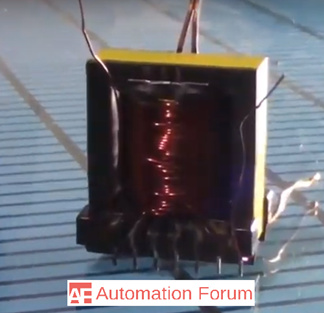# Why do we need a transformer and what is a pulse transformer

What is a transformer

A transformer can be considered as the backbone of electrical power distribution. In the presence of constant magnetic flux, an AC transformer is a basic component that transforms an AC power at one voltage to another voltage. It contains more than one coils of conducting wire that is enfolded in a mutual core. These conducting coils are not electrically attached to each other. The only connection among them is they share magnetic flux present within the core.

Why do we need a transformer

An AC transformer transforms one AC voltage to another voltage without changing the main power provided to it. A transformer increases the output voltage in a particular case, in the same condition it reduces the rated current to maintain the power into the circuit equal to the output power from it. AC power transmission is done with the help of a transformer its voltage will boost up to transmit for long distance at negligible power losses, and its voltage is decreased for some other application. The line losses in a power system are directly related to the square of the current present in the transmission line. If we increase the amount of the transmission voltage level then it will reduce the transmission current by ten times. So without the transformer, it is not possible to utilize electrical power in different ways as we are using it currently.

What is a pulse transformer

The magnetic flux of a pulse transformer core doesn’t alternate between the positive and negative values like in the AC transformer, the operation mode of pulse transformer is the unipolar mode. A biasing DC magnetic field is created in the transformer core by a fixed DC current, and it would help the field to cross the zero line. Pulse transformer mostly operates at high frequency necessitating the use of low loss cores. Pulse transformer can be divided into power and signal transformer an example of a power pulse transformer application would be precise control of a heating element from a fixed DC voltage source. The voltage is stepped up and down as needed by the pulse transformer’s turn ratio. By using a switch the pulse transformer power can be turned off and on, at an operating frequency and pulse duration which would deliver the required power.

The performance of a pulse transformer is depended on the pulse voltage, voltage ratio, pulse duration, power or impedance level, droop on top, and type of the load.What are the types of pulse transformer

Pulse transformer can be divided into pulse power and pulse signal transformer. Pulse transformer provides electrical isolation between the input and the output. The transformer which is used in forwarding converter power supplies is essentially a power type pulse transformer. There is also a high power pulse transformer which has a power capacity of more than 500Kilowatts. The signal type pulse transformer mostly does the delivery of the signal at the output. This transformer will deliver a pulse-like signal or a series of pulses. In order to adjust the signal amplitude and to attain the impedance matching between the source and the load, the turn ratio of the pulse transformer can be used. Pulse transformers are often used for the transmission of the digital data and in the gate drive circuitry of the transistors. The design of pulse transformer changes according to the power rating, inductance, voltage level, operating frequency, size, and impedance.

Where are pulse transformers used

Signal type pulse transformers are used in digital logic and telecommunication circuits, it is often used for matching logic drives to transmission lines. Pulse transformers are used in power control circuits like camera flash controllers. These transformers are used in the electrical energy distribution process, it does the interface of low voltage control circuitry to the high voltage gates of power semiconductors.

What are the advantages of pulse transformer

Pulse transformer can be used to operate at high frequencies, these transformers are compact and it can transfer more power than the same size normal transformer. The pulse transformer core has higher quality due to the Foucault current at high frequency.